The fundamental (basic) identities discussed in the previous section involved only one variable. The following identities, involving two variables, are called trigonometric addition identities.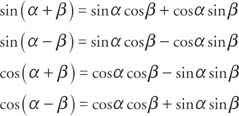These four identities are sometimes called the sum identity for sine, the difference identity for sine, the sum identity for cosine, and the difference identity for cosine, respectively. The verification of these four identities follows from the basic identities and the distance formula between points in the rectangular coordinate system. Explanations for each step of the proof will be given only for the first few examples that follow.

Example 1: Change sin 80° cos 130° + cos 80° sin 130° into a trigonometric function in one variable (Figure 1).Figure 1
Drawing for Example 1.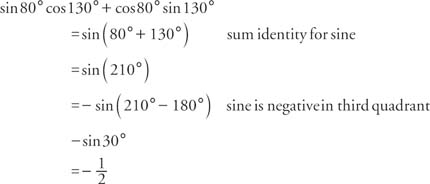Additional identities can be derived from the sum and difference identities for cosine and sine.

Example 2: Verify that cos (180° − x) = − cos xExample 3: Verify that cos (180° + x) = − cos xExample 4: Verify that cos (360° − x) = cos x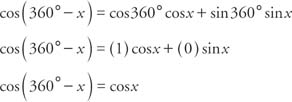The preceding three examples verify three formulas known as the reduction formulas for cosine. These reduction formulas are useful in rewriting cosines of angles that are larger than 90° as functions of acute angles.

Example 5: Verify that sin (180° − x) = sin xExample 6: Verify that sin(180° + x) = − sin xExample 7: Verify that sin (360° − x) = − sin xThe preceding three examples verify three formulas known as the reduction formulas for sine. These reduction formulas are useful in rewriting sines of angles that are larger than 90° as functions of acute angles.

To recap, the following are the reduction formulas (identities) for sine and cosine. They are valid for both degree and radian measure.Example 8: Verify that sin 2 x = 2 sin x cos x.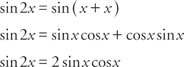Example 9: Write cosβcos(α − β) − sinβsin(α − β) as a function of one variable.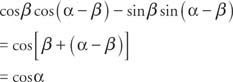Example 10: Write cos 303° in the form sinβ, where 0 <β< 90°.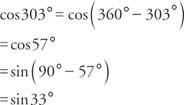Example 11: Write sin 234° in the form cos 0 <β < 90°.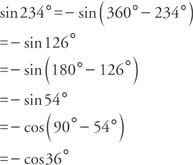Example 12: Find sin (α + β) if sin (α + β) if sin α =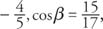and α and β are fourth quadrant angles.

First find cos α and sin β. The sine is negative and the cosine is positive in the fourth quadrant.